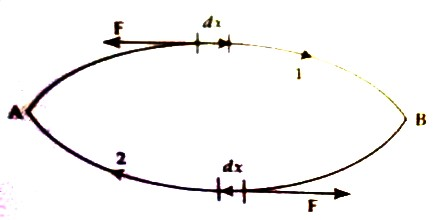# Non-conservative Force

Non-conservative Force

A force is said to be non-conservative if the work done by the force depends on the path along which the body moves. Work done by such a force over a round trip is not zero. Examples – Frictional force, viscous force etc.

Characteristics of non-conservative force:

(1) This force does not depend on the position only

(2) Work done by this force depends on the path in transferring a body from one place to another place

(3) Work done by the non-conservative force cannot be regained completely.

(4) Non-conservative force does not follow the principle of conservation of mechanical energy.Let an object be pushed from point A to point B along the smooth horizontal floor through path 1 [Figure]. In this case, frictional force acts against the motion of the object. So, in this transfer work is to be done against the frictional force; because frictional force is always a force-resisting motion. Let a small segment of displacement of motion be dx and this displacement be against the frictional force F, then work done,

dW = – Fdx

Total work done in taking the object through path 1 from A to B is the summation of all the small work done, i.e.,

W1 = – ∫1 Fdx

Now, if the object is again taken from B to A along the path 2, the frictional force in this case also will act against the motion. So,

W2 = – ∫2 Fdx

In both cases since work is done against frictional force, both work will be negative and their summation will not be zero.

Now, total work done,

W = W1 + W2 = – ∫1 Fdx – ∫2 Fdx ≠ 0

So, work done by frictional force is not recoverable. Hence, frictional force is non-conservative force.

According to the characteristics of conservative and non-conservative forces it can be shown that,

To raise a body to a height It against the gravitational force F the amount of work done = – Fh. Now, if it is released from that height to ground, then work done will be + Fh.

So, in raising a body to a height ‘h’ and in bringing back to the ground work done will be (— Fh + Fh = 0) zero. Hence, gravitational force is conservative. Similarly, electric force, magnetic force etc are conservative force.

On the other hand, in case at fraction, frictional force resists the motion of a moving body. So work done by it on the body becomes negative. So, frictional force is a non-conservative force.